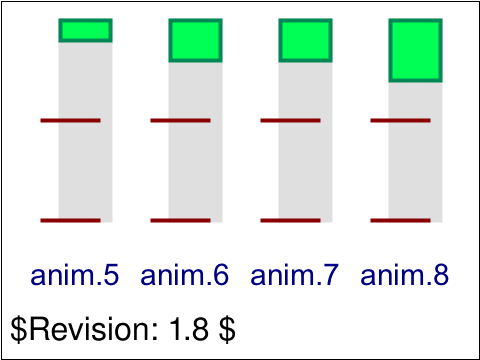SVG Image PNG Image FAIL#### Pass Criteria

The test has passed if the four green rectangles each animate their height according to the following:

The leftmost rect:

• when the animation starts the height of the green rect should make it align with the bottommost red indicator line
• after two seconds the height should jump to be 10% of the height of the gray rect it overlaps
• after four seconds the height should jump to make the green rect align with the bottommost red indicator line
• after six seconds the height should jump to its final position, 10% of the height of the gray rect

The next to leftmost rect:

• when the animation starts the height of the green rect should be 110% of the height of the gray rect
• after two seconds the height should jump to be 20% of the height of the gray rect
• after four seconds the height should jump to be 110% of the height of the gray rect
• after six seconds the height should jump to its final position, 20% of the height of the gray rect

The next to rightmost rect:

• when the animation starts the height of the green rect should make it align with the bottommost red indicator line
• after two seconds the height should jump to be 10% of the height of the gray rect
• after four seconds the height should jump to be 110% of the height of the gray rect
• after six seconds the height should jump to its final position, 20% of the height of the gray rect

The rightmost rect:

• when the animation starts the height of the green rect should be 110% of the height of the gray rect
• after two seconds the height should jump to be 20% of the height of the gray rect
• after four seconds the height should jump to be 120% of the height of the gray rect
• after six seconds the height should jump to its final position, 30% of the height of the gray rect